• matlab局部加权线性回归
千次阅读
2017-03-17 11:00:06

# 局部加权线性回归（LWLR）

对于线性回归算法，容易出现欠拟合，而多项式回归又容易出现过拟合。因此出现了局部加权回归

## 模型

y(i)=θTx(i)

和线性回归的模型相同，但是对于每一个预测点， θ $\theta$都需要重新计算，并不是固定不变的。

## 损失函数

L(θ)=12Mi=1mwi(yiθTxi)2

wi=exp(|xix|22k2)

对于预测点x, wi $w_i$表示 xi $x_i$与x的距离，如果两者距离近，则 wi=1 $w_i=1$ ,如果两者较远，则 wi=0 $w_i=0$。k则控制 wi $w_i$的衰减快慢。所以损失函数可以理解为对预测点附近样本点进行线性回归运算。

## 算法

局部线性加权算法需要对每一个预测样本点重新计算权值。

### 1.梯度下降

我们的优化目标是：

minθL(θ)

Lθ=1Mi=1mwi(yiθTxi)xi=1MXTw1(yiθTxi)wM(yiθTxM)=1MXTW(YXθ)

因此迭代公式为

θ:=θλ1MXTW(XθY)

其中W为斜对角矩阵

### 2.解析法

L(θ)θ=0

θ=(XTWX)1XTWY

## 评价

1. 局部加权回归在每一次预测新样本时都会重新的确定参数，从而达到更好的预测效果。
2. 对于局部加权线性回归算法，每次进行预测都需要全部的训练数据（每次进行的预测得到不同的参数θ），没有固定的参数θ，所以是非参数算法。
3. 当数据规模比较大的时候计算量很大，学习效率很低。并且局部加权回归也不是一定就是避免underfitting。
4. 非参数学习算法，每进行一次预测，就需要重新学习一组 ， 是变化的，所以需要一直保留训练样本。也就是说，当训练集的容量较大时，非参数学习算法需要占用更多的存储空间，计算速度也较慢。
更多相关内容
• 局部加权线性回归，使用matlab编写，有结果图 程序流程为 标准化 样本矩阵与输出向量 计算权重对角矩阵 梯度下降 反标准化 显示
• 局部加权线性最小二乘就不需要我们预先知道待求解的模型，因为该方法是基于多个线性函数的叠加，最终只用到了线性模型。计算线性模型时引入了一个加权函数： 来给当前预测数据分配权重，分配机制是：给距离近的点更...

通常我们使用的最小二乘都需要预先设定一个模型，然后通过最小二乘方法解出模型的系数。

而大多数情况是我们是不知道这个模型的，比如这篇博客中z=ax^2+by^2+cxy+dx+ey+f 这样的模型。

局部加权线性最小二乘就不需要我们预先知道待求解的模型，因为该方法是基于多个线性函数的叠加，最终只用到了线性模型。

计算线性模型时引入了一个加权函数：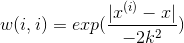来给当前预测数据分配权重，分配机制是：给距离近的点更高的权重，给距离远的点更低的权重。

公式中的k类似与高斯函数中的sigma。

当sigma变大时，函数变得矮胖，计算局部线性函数时更多的使用全局数据；

当sigma变小时，函数变得瘦高，计算局部线性函数时更多的使用局部数据。

代码如下：

clear all;

close all;

clc;

x=(1:0.1:10)';

y=x.^2+x+3 +rand(length(x),1)*6;

plot(x,y,'.')

sigma=0.1; %设置局部窗口，越大越使用全局数据，越小越使用局部数据

W=zeros(length(x));

C=[];

for i=1:length(x)

for j=1:length(x)

W(j,j)=exp(-((x(i)-x(j))^2)/(2*sigma^2)); %权重矩阵

end

XX=[x ones(length(x),1)];

YY=y;

C=[C inv(XX'*W*XX)*XX'*W*YY]; %加权最小二乘，计算求得局部线性函数的系数

end

re=diag(XX*C);

hold on;

plot(x,re);

结果如下：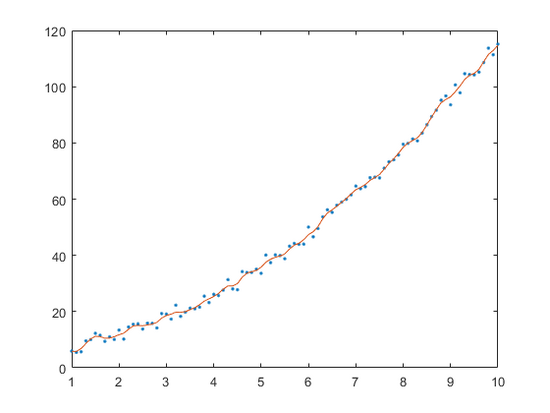可以看出，红色的局部线性函数最终拟合出了全局的数据。

不过该方法既然不需要知道模型，那我们如何预测未来的数据结果呢？

展开全文• 局部加权线性拟合相对于多项式拟合，计算线性模型时引入了一个加权函数： 来给当前预测数据分配权重。给距离近的点更高的权重，给距离远的点更低的权重，往往能具有更高的精确性。 x=(1:0.1:10)'; y=x.^2+3.*x+...

局部加权线性拟合相对于多项式拟合，计算线性模型时引入了一个加权函数：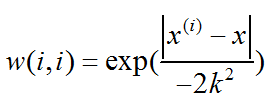来给当前预测数据分配权重。给距离近的点更高的权重，给距离远的点更低的权重，往往能具有更高的精确性。

x=(1:0.1:10)';
y=x.^2+3.*x+2 +rand(length(x),1)*8;
sigma=0.1;              %设置局部窗口，越大越使用全局数据，越小越使用局部数据
W=zeros(length(x));
C=[];
for i=1:length(x)
for j=1:length(x)
W(j,j)=exp(-((x(i)-x(j))^2)/(2*sigma^2));   %权重矩阵
end
XX=[x ones(length(x),1)];
YY=y;
C=[C inv(XX'*W*XX)*XX'*W*YY];   %加权最小二乘，计算求得局部线性函数的系数
end
subplot(1,2,1);
plot(x,y,'.');
re=diag(XX*C);
hold on;
plot(x,re);
title('局部加权线形拟合');
subplot(1,2,2);
plot(x,y,'.');
hold on
p=polyfit(x,y,3);
yi=polyval(p,x);
plot(x,yi);
title('最小二乘多项式拟合');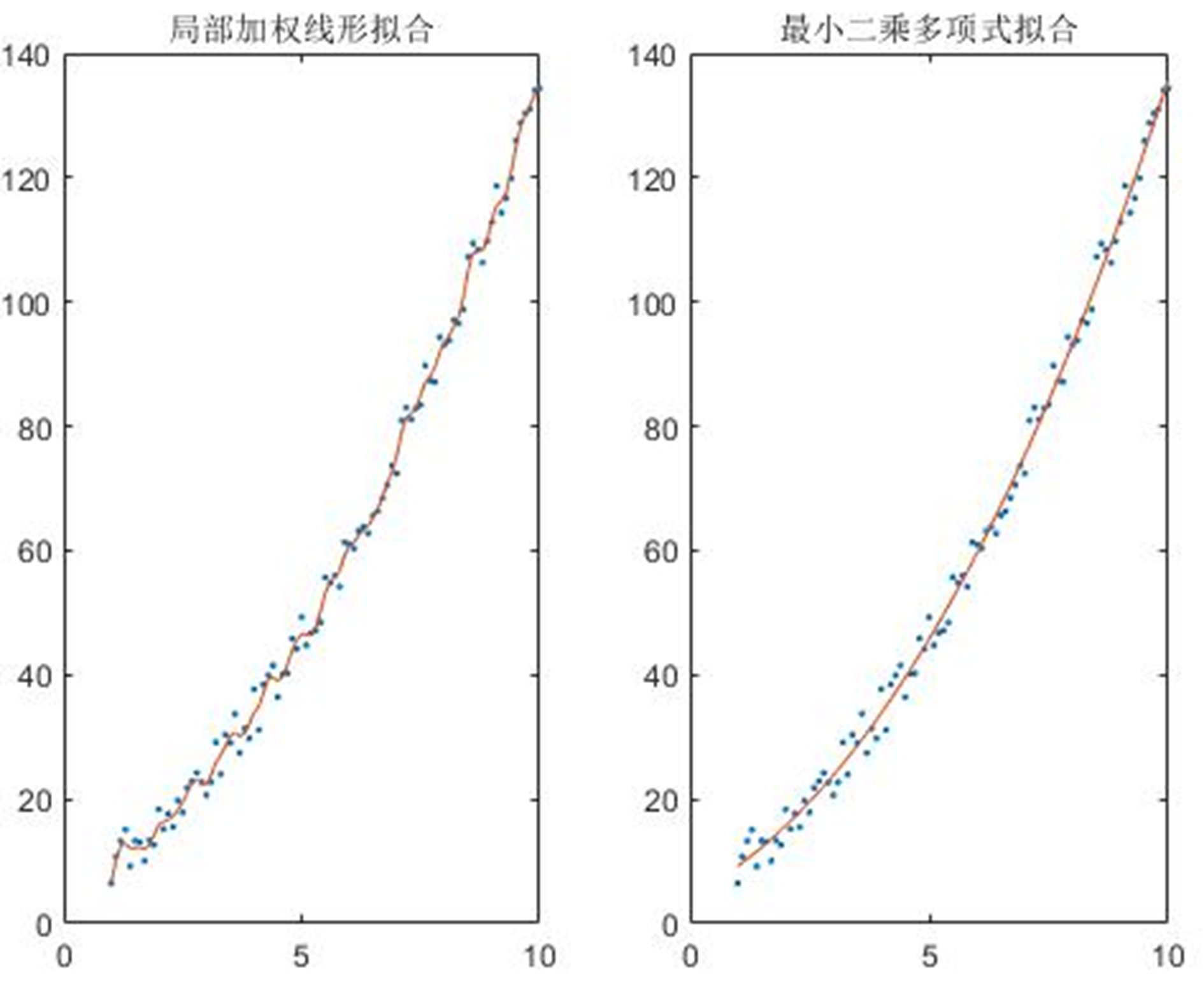作者：QinL

展开全文• 文章描述了局部加权线性回归基本原理（英文），并且用MATLAB上实现了对一个机器人不规则路径的非线性拟合拟合。文档末尾附有代码。
• 回归的目的是预测数值型的目标值，其中最简单的方法是线性回归，线性回归意味着可以将输入项分别乘以一些常量，再将结果加起来得到输出。... 其中的一个方法是局部加权线性回归（Locally Weighted...

回归的目的是预测数值型的目标值，其中最简单的方法是线性回归，线性回归意味着可以将输入项分别乘以一些常量，再将结果加起来得到输出。但是，线性回归的一个问题是有可能出现欠拟合现象，因为它求的是具有最小均方误差的无偏估计。如果模型欠拟合将不能取得最好的预测效果。所以有些方法允许在估计中引入一些偏差，从而降低预测的均方误差。

其中的一个方法是局部加权线性回归（Locally Weighted Linear Regression,LWLR）。在该算法中，我们给待预测点附近的每一个点赋予一定的权重，然后在这个子集上基于最小均方误差来进行普通的回归。与kNN一样，这种算法每次预测均需事先选取出对应的数据子集。该算法解出回归系数w的形式如下：

其中，w是一个矩阵，用来给每个数据点赋予权重。

LWLR使用“核”（与支持向量机中的核类似）来对附近的点赋予更高的权重。核的类型可以自由选择，最常用的核就是高斯核，高斯核对应的权重如下：

这样就构建了一个只含对角元素的权重矩阵w，并且点x与x(i)越近，w(i,i)将会越大。上述公式包含一个需要用户指定的参数k，它决定了对附近的点赋予多大的权重，这也是使用LWLR时唯一需要考虑的参数，参数k与权重的关系如下图所示：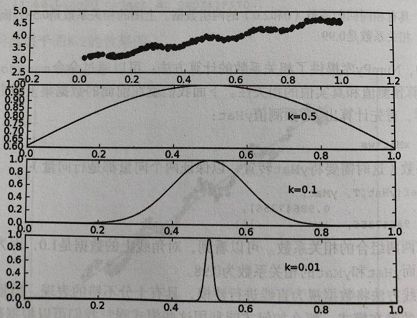使用局部加权线性回归进行数据拟合，用MATLAB代码如下：

clear ;
close all;
% x = [1:50].';
% y = [4554 3014 2171 1891 1593 1532 1416 1326 1297 1266 ...
%     1248 1052 951 936 918 797 743 665 662 652 ...
%     629 609 596 590 582 547 486 471 462 435 ...
%     424 403 400 386 386 384 384 383 370 365 ...
%     360 358 354 347 320 319 318 311 307 290 ].';
len=90;
y=data(:,8);
y=y(1:len,1);
x=1:len;
x=x.';

m = length(y); % store the number of training examples
x = [ ones(m,1) x]; % Add a column of ones to x
n = size(x,2); % number of features
theta_vec = inv(x'*x)*x'*y;
tau = [ 1 10 25 ];
y_est = zeros(length(tau),length(x));
for kk = 1:length(tau)
for ii = 1:length(x);
w_ii = exp(-(x(ii,2) - x(:,2)).^2./(2*tau(kk)^2));
W = diag(w_ii);
theta_vec = inv(x'*W*x)*x'*W*y;
y_est(kk, ii) = x(ii,:)*theta_vec;
end
end

figure;
plot(x(:,2),y,'ks-'); hold on
plot(x(:,2),y_est(1,:),'bp-');
% plot(x(:,2),y_est(2,:),'rx-');
% plot(x(:,2),y_est(3,:),'go-');
legend('measured', 'predicted, tau=1', 'predicted, tau=10','predicted, tau=25');
grid on;
xlabel('Page index, x');
ylabel('Page views, y');
title('Measured and predicted page views with weighted least squares');

效果如图所示：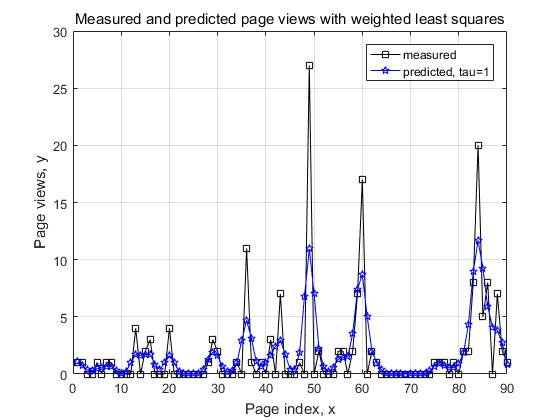用局部加权线性回归进行数据预测，结果如图所示：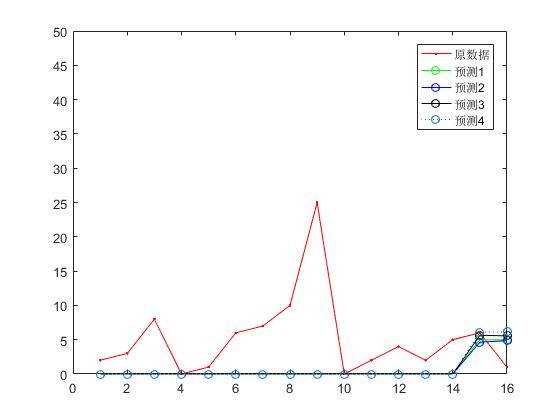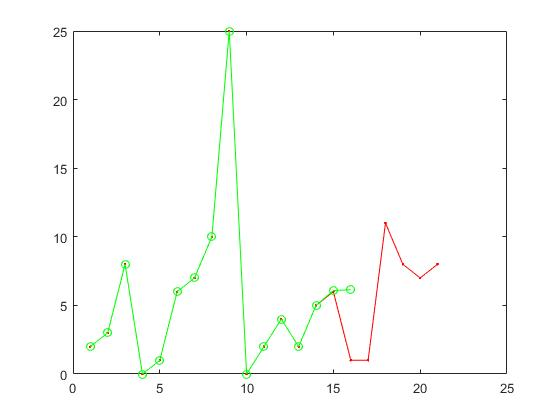展开全文• 一，单变量线性回归：1. 数据分布，x轴是属性城市人口，y轴是标签值盈利：2. 目的：使用一个线性函数去拟合上面这些数据；该线性函数如下只有两个参数，利用梯度下降找出使损失值最小时，对应的两个参数值，即得到了...
• 局部加权投影回归（LWPR）是一种完全增量的在线算法，用于在高维空间中进行非线性函数逼近，能够处理冗余和不相关的输入维。 它的核心是使用局部线性模型，该模型由输入空间中选定方向上的少量单变量回归所跨越。 ...开源软件
• 利用局部加权回归，对UCI数据进行分段线性拟合，使用时需修改下路径名。
• 请注意最后四个残差的线性趋势，这表明模型可能不会随着 x 增加而快速增加。而且，残差的模随 x 增大而减小，这表明测量误差可能取决于 x。这些特性值得进一步研究，但由于数据点太少，不足以确定这些明显模式的研究...
• 函数 fLOESS 对一维数据执行 LOESS（使用二阶多项式的局部加权非参数回归拟合）平滑，无需 Matlab 曲线拟合工具箱。 这可能被认为是 LOWESS 的一种稍微更好的方法，它使用线性拟合产生局部加权回归
• LOWESS- 局部加权散点图平滑，不需要 matlab 中的统计工具箱。 此回归将适用于 X 和 Y 之间的线性和非线性关系。 修改： 12/19/2008 - 添加上下 LOWESS 平滑。 这些额外的平滑显示了 Y 的分布如何随 X 变化。这些...
• matlab开发-线性和非线性数据增强的低局部加权散射图平滑。LOWESS，像LOWESS这样的稳健回归允许检测趋势，否则方差太大
• ## 局部加权线性回归

千次阅读 2017-03-10 11:00:44
换成线性代数的表述方式： 是mxm维的对角矩阵 是mxn维的输入矩阵 是mx1维的结果 是nx1维的参数向量 令 有 既 权重定义为： 参数τ控
• 换成线性代数的表述方式： 是mxm维的对角矩阵 注意这个地方对角线处,并不是指次数,而是指w的序号. 是mxn维的输入矩阵 是mx1维的结果 是nx1维的参数向量 令 有 既 这里的θ就是书上的w 权重...
• STANFORD机器学习课程（Andrew Ng主讲），第3课学习笔记。在本节中，介绍局部加权线性回归算法（Loess/LWR）和Logistic回归算法。机器学习 Logistic 最小二乘法
• 根据《机器学习实战》中的phthon代码改编而来，详细说明参见原书，测试数据都是用的原书数据 主程序：lwlr_test.m clc; clear; %高斯核波长 k=0.01; %读取数据， fid=fopen('ex0.txt','r');... linei数据挖掘 机器学习
• ## 断点分析法_局部线性回归_matlab

万次阅读 热门讨论 2016-07-11 10:51:58
本文介绍断点分析法在数据作假方面的应用 局部线性回归matlab实现断点分析法 局部线性回归 核函数
• 吴恩达机器学习（四）局部加权回归...另外，局部加权回归可以用线性回归的方法得到一个非线性的拟合结果。这句话有点奇怪，但了解局部加权回归的原理就能明白其中的原因了。 局部加权回归的原理 相关参数定义： m...机器学习
• 回归预测 | MATLAB实现RBF径向基神经网络非线性函数回归神经网络 RBF 径向基神经网络
• 关于非参数和参数方法的区别，就是在分析之前有没有对预测做一些限制，比如认为特征和响应变量之间具有线性关系，可以通过线性方程拟合，我们只需要求出方程的系数就是参数方法，比如之前提到的线性回归、多项式回归...
• 目前，回归诊断不仅用于一般线性模型的诊断，还被逐步推广应用于广义线性模型领域（如用于logistic回归模型），但由于一般线性模型与广义线性模型在残差分布的假定等方面有所不同，所以推广和应用还存在许多问题。...R语言 Lowess
• 换成线性代数的表述方式：3 4是mxm维的对角矩阵 5是mxn维的输入矩阵 6是mx1维的结果 7是nx1维的参数向量 8 9 令11 有12 既13 权重定义为：14 参数τ控制权重函数的宽度，τ越大，权重函数越宽： 15 下面...机器学习
• 轮廓平滑是通过将所有轮廓点投影到局部回归线上来完成的。 对于每个点，位于轮廓上的 N 个相邻点在每一侧采样，并计算局部回归线。 然后当前点投影在这条线上。 将此算法应用于所有点可以平滑轮廓，并在某种程度上...
• 逻辑回归与线性回归案例的python及matlab实现（包括基于线性回归的利润预测案例，局部加权回归）
• 线性回归理论推导 波士顿房价预测 matlab2021实现 python实现 前言 包含线性回归的梯度下降与正规方程的理论推导。 波士顿房价预测示例 matlab2021a版本 + juptype python版本 全部的内容如下:因为无法上传...机器学习 深度学习 python...

# matlab局部加权线性回归matlab 订阅